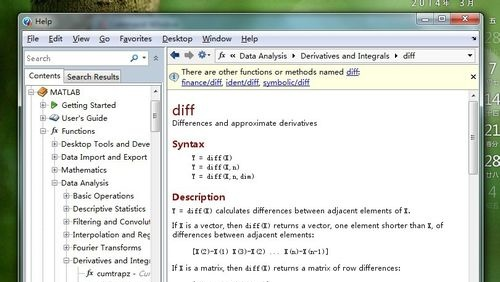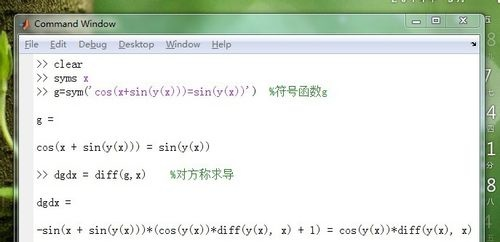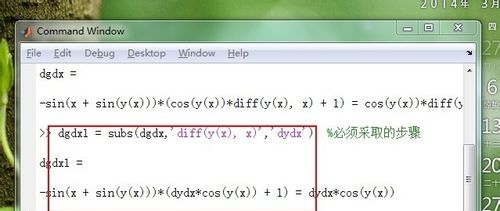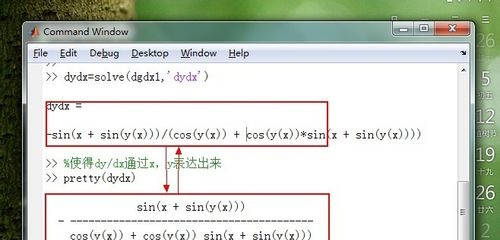• matlab隐函数求解
千次阅读
2020-11-14 22:29:28

Matlab 隐函数方程求解&最小二乘法拟合一阶线性拟合&二阶拟合&传感器实验python matlab 机器学习
更多相关内容
• A=[sinxcosy, e^siny], B=[siny,1+e^(x/y)]C=sqrt(A*B')-1C(x,y)=0为隐函数解：fzero是求一元方程根的函数，后面的1是初值，你也可以尝试其他的初值。"@ (x) @ (y)表达式"是构造了一个双重匿名函数，传入x后就相当于...

1.

fzero()

2. fsolve()+ inline()

3. solve()

4. 先求解析解，后用替代法求数值解。

A=[sinxcosy, e^siny], B=[siny,1+e^(x/y)]

C=sqrt(A*B')-1

C(x,y)=0为隐函数

解：fzero是求一元方程根的函数，后面的1是初值，你也可以尝试其他的初值。"@ (x) @ (y)

表达式"是构造了一个双重匿名函数，传入x后就相当于一个关于y的一元函数了。关于这方面的详细讨论本人今年5、6月份将要出版的书：《MATLAB高效编程技巧与应用-30个案例分析》里有涉及。

这本质上就是一个参数传递的问题，匿名函数可以方便的实现参数传递，再复杂的还可以通过嵌套函数实现。就拿你5楼举的例子来说，起码有两个方法可以很好的解决：一个就是还用我4楼给你说的方法，只不过是构造两个匿名函数：

代码：

A =

@(x,y) [sin(x)*cos(y), exp(sin(y))];B = @(x,y)

[sin(y),1+exp(x/y)];

C = @(x) @(y) sqrt(A(x,y)*B(x,y)')-1;

Y = @(x) fzero(C(x),1)

Y =

@(x)fzero(C(x),1)

>> Y(1)

ans =

-8.4211e-018

>> Y(2)

ans =

-6.4283e-016

>> Y(3)

ans =

-7.2065e-016

还有一种就是先用符号计算把C的符号表达式算出来，然后再生成匿名函数：

代码：

syms x y

A = [sin(x)*cos(y),

exp(sin(y))];B

= [sin(y),1+exp(x/y)];

C = sqrt(A*B')-1;

eval_r(['fun = @(x) @(y)' ,char(C)]);

Y = @(x) fzero(fun(x),1)

fun =

@(x)@(y)(exp(sin(y))*(exp(conj(x)/conj(y))+1)+sin(conj(y))*cos(y)*sin(x))^(1/2)-1

Y =

@(x)fzero(fun(x),1)

>> Y(1)

ans =

-8.4211e-018

>> Y(2)

ans =

-6.4283e-016

>> Y(3)

ans =

-7.2065e-016

关于用MATLAB求解隐函数的问题

用MATLAB求解一个隐函数，x为待求值，其余均为已知参数，函数为(a+b*cos(c)-d*cos(e))/cos(x)=(f+b*sin(c)-d*sin(e))/sin(x)

解：如果Matlab 装了Symbolic

Toolbox的话，Matlab可以直接求解析解，如下：

solve('(a+b*cos(c)-d*cos(e))/cos(x)=(f+b*sin(c)-d*sin(e))/sin(x)',

'x')

ans =

-(log((a*exp(c*i)*exp(e*i) + b*exp(2*c*i)*exp(e*i) -

d*exp(c*i)*exp(2*e*i) + f*exp(c*i)*exp(e*i)*i)/(b*exp(e*i) -

d*exp(c*i) + a*exp(c*i)*exp(e*i) -

f*exp(c*i)*exp(e*i)*i))*i)/2

用subs函数可以将solve接出来的解析解里面的变量用数字替换掉，然后得到数值解。

举个简单的例子，求a*x=b的解，先求解析解，然后用a=2，b=1代入求出数值解：

>> solution=solve('a*x=b', 'x')

solution =

b/a

>> num_solution = subs(solution,

'[a,b]', [2 1])

num_solution =

1/2

>> num_solution =

subs(num_solution)

num_solution =

0.5000

qi=solve('qi-bet*detm/(bet/(B*0.018526^0.2*qi^0.6/di^0.6)+0.7*10^(-3)*2*ft/(ft+fi)/203+r0+1/aj)=0','qi')

其中除了qi外，其他参数的值都在前边的程序中算出来了，不知道是哪里出错了，运行总是出现Error using

==> solve

'

qi-bet*detm/(bet/(B*0.018526^0.2*qi^0.6/di^0.6)+0.7*10^(-3)*2*ft/(ft+fi)/203+r0+1/aj)=0

' is not a valid expression or equation.

不知道是不是solve不能调用前面的值，如何解决??

问题补充：

参数是和我输入的变量值有关的，会变化的，或者有没有其他的办法来解这个方程。

解：

>> a=3;

>> solve('a*x-3=0','x')

得到的结果是

ans =

3/a

也就是说，solve里面将a当成符号，而没有把你a=3代入。

试着改成

>> subs(solve('a*x-3=0','x'))

就得到ans=1的结果了。

你的问题也可以这样弄。

solve再加一个subs()

另外你也可以自己将方程中的变量先将值代入，然后再解方程

比如：

clear

>> a=3;

>> equ=sprintf('a*x-3=0');

>> equ=subs(equ); %这一步就是将a=3代入

>> solve(equ,x)

注意：

function y=fcn(a,b) %u is a vector

S=solve('a+b/r-z=1','r+z=38','r','z');

y=[S.r;S.z];//Embedded matlab function中得到多个变量值的方法

展开全文隐式函数matlab
• 问题描述：MATLAB复杂隐函数求解!求大神指导!syms D1 D2 G1 G2 al E1 E2 Km w6G1=10^(-5);G2=10^(-5); E1=2*(10^11);E2=2*(10^11);Km=1.2;w6=2*(10^9);for D1=1.3:0.1:1.8;for D2=1.3:0.1:1.8;ac1=(G1^2)/((Km*0.01/...

问题描述：

MATLAB复杂隐函数求解!求大神指导!

syms D1 D2 G1 G2 al E1 E2 Km w6

G1=10^(-5);G2=10^(-5); E1=2*(10^11);E2=2*(10^11);Km=1.2;w6=2*(10^9);for D1=1.3:0.1:1.8;

for D2=1.3:0.1:1.8;

ac1=(G1^2)/((Km*0.01/2)^(2/(D1-1)));

ac2=(G2^2)/((Km*0.01/2)^(2/(D2-1)));

w=2*(pi^(1/2))*E1*(G1^(D1-1))*D1*(al^(D1/2))/3;

r=(2/(3-2D1))*(al^((3-2D1)/2)-ac1^((3-2D1)/2))-((al^((2-D2)/2))*2/(5-2D1-D2)*(al^((5-2D1-D2)/2)-ac1^((5-2D1-D2)/2)));

t=Km*w6*D1*(al^(D1/2))/2;

u=(2/(2-D1)*(ac1^((2-D1)/2))-((al^((2-D2)/2)))*2/(4-D2-D1)*(ac1^((4-D2-D1)/2)));

P2=w*r+t*u;

w1=2*(pi^(1/2))*E2*(G2^(D2-1))*D1*(al^((D1+D2-2)/2))/3;

r1=2/(5-2D1-D2)*(al^((5-2D1-D2)/2)-ac2^((5-2D1-D2)/2));

t1=Km*w6*D1*(al^((D1+D2-2)/2))/2;

u1=2/(4-D2-D1)*(ac2^((4-D2-D1)/2));

P1=w1*r1+t1*u1;

P=P1+P2;P=3*10^4;

%想在已知P和D1,D2的情况下求解al,D1和D2是一组数据,最后画出三维图

1个回答

分类：

数学

2014-12-16

问题解答：

我来补答

题目写的比较乱.明确几点：1、是不是说对于D1=1.3:0.1:1.8,D2=1.3:0.1:1.8的每一种组合,按照P=P1+P2=3*10^4来求解a1?2、对于这样的复杂方程,求解析解是不太现实的,应该求数值解.3、方程可能存在多个解,怎么处理?例如,取D1=D2=1.5,画出P-3E4的曲线如下：显然,在0-30范围内有两个解.

再问： 你好，谢谢你的回答。以上函数中的al在我的设定中是有一定物理意义的，应该是个小于1大于0的正数。是否能够给出：在已知P的值时，得出D1，D2，al的三维关系图。麻烦大神把程序传上来！我快被这事弄崩溃了！

再答： 参考代码：syms D1 D2 G1 G2 al E1 E2 Km w6

G1=10^(-5);G2=10^(-5); E1=2*(10^11);E2=2*(10^11);Km=1.2;w6=2*(10^9);

[DD1,DD2] = meshgrid(1.3:0.1:1.8);

A1 = DD1*NaN;

for ii=1:size(DD1,1)

for jj=1:size(DD2,2)

D1 = DD1(ii,jj);

D2 = DD2(ii,jj);

ac1=(G1^2)/((Km*0.01/2)^(2/(D1-1)));

ac2=(G2^2)/((Km*0.01/2)^(2/(D2-1)));        (因字数限制，此部分代码不变，删除)

t1=Km*w6*D1*(al^((D1+D2-2)/2))/2;

u1=2/(4-D2-D1)*(ac2^((4-D2-D1)/2));

P1=w1*r1+t1*u1;

P=P1+P2;

try

A1(ii,jj)=fzero(@(a1)subs(P-3E4,a1),[eps 1]);

catch

fprintf('Error: D1=%g, D2=%g, %s\n',D1,D2,lasterr)

end

end

end

mesh(DD1,DD2,A1)

view(3)

‍‍其中，当D1=1.3, D2=1.4时，无解(用ezplot(P-3E4,[0 1])容易看到，曲线始终位于实轴之下)。

再答： 参考代码：syms D1 D2 G1 G2 al E1 E2 Km w6

G1=10^(-5);G2=10^(-5); E1=2*(10^11);E2=2*(10^11);Km=1.2;w6=2*(10^9);

[DD1,DD2] = meshgrid(1.3:0.1:1.8);

A1 = DD1*NaN;

for ii=1:size(DD1,1)

for jj=1:size(DD2,2)

D1 = DD1(ii,jj);

D2 = DD2(ii,jj);

ac1=(G1^2)/((Km*0.01/2)^(2/(D1-1)));        (此处有部分代码无改动，因字数限制省略)

u1=2/(4-D2-D1)*(ac2^((4-D2-D1)/2));

P1=w1*r1+t1*u1;

P=P1+P2;

try

A1(ii,jj)=fzero(@(a1)subs(P-3E4,a1),[eps 1]);

catch

fprintf('Error: D1=%g, D2=%g, %s\n',D1,D2,lasterr)

end

end

end

mesh(DD1,DD2,A1)

view(3)

注意，有些情况下(例如D1=1.3, D2=1.4)方程无解。

展开全文阅读

展开全文• PAGE / NUMPAGES 小辈求解隐函数 其中x 和y为自变量和因变量别的参数值已经给出具体程序如下 syms x y; k0=4.0516e+06; em=-104+3.68i; ed=2.152089; f=em*k0*sqrt(y^2-ed*tanh(sqrt(y^2-ed*k0*x/2/(10^9)+ed*k0*...
• 隐函数求导一般都比较繁琐，下面介绍一下如何用matlab隐函数求导，这样一来我们就可以解放双手，轻松求解隐函数的导数。工具/材料matlab软件正常运行电脑操作方法01matlab求导指令diff简介：这个函数是单词...

隐函数求导一般都比较繁琐，下面介绍一下

如何用matlab对隐函数求导，这样一来我们就可以解放双手，轻松求解隐函数的导数。工具/材料

matlab软件

正常运行电脑

操作方法

01

matlab求导指令diff简介：

这个函数是单词differential(微分)的简写，用于计算微分，实际上计算的是差商，可以计算一阶导数的有限差分近似。diff指令的具体用法(调用语法)如下：

diff(函数),求的一阶导数；

diff(函数,n),求的n阶导数(n是具体整数)；

diff(函数,变量名),求对的偏导数；

下面是

doc diff的帮助信息：02

对隐函数求导，下面就用matlab求一下隐函数cos(x+siny)=siny的导数。具体的代码及计算结果如下图所示：03

用新的变量名dydx替代dgdx中的diff(y(x), x)，具体的代码及计算结果如下图所示：04

对变量dgdx1代表的符号方程关于dydx的求解，使得dy/dx通过x，y表达出来。具体的代码及计算结果如下图所示：05

注意：

1.生成隐函数的指令中y必须写成y(x)，表明y是x的函数。

2.第三步中把dgdx变量所包含的diff(y(x), x)字符串替换为dydx，这是必须的步骤，因为diff(y(x), x)不能作为求解的变量名使用。

好了，以上就是大致内容了，(END)

声明：该文观点仅代表作者本人，天晴资讯网系信息发布平台，仅提供信息存储空间服务。

展开全文• 如果对MATLAB无感，可以用1stopt，代码更简单：Title "Type your title here";Parameters x[0.32,0.65],y[0.59,1.22],z;StepX = 20;StepY = 20;PlotFunction 3/4/(sqrt(z))+(2-x/y)-3*x*z/y/(sqrt(y^2-3*z^2))=0;...
• matlab求解1隐函数小辈求解隐函数， 其中x 和y为自变量和因变量。别的参数值已经给出，具体程序如下：syms x y;k0=4.0516e+06;em=-104+3.68i;ed=2.152089;f=em*k0*sqrt(y^2-ed)*tanh(sqrt(y^2-ed)*k0*x/2/(10^9))+...
• 问题描述 已知条件如下： ①隐函数H(x,y1) =exp(2*x-y1)*log(x-3*y1)-4 = 0 ...我们需要借助matlab的官方函数fimplicit绘制隐函数H和M的图像 对于隐函数H(x,y1)的图像，可以得到一组一一对应的x，y1 ......matlab 隐函数
• 类似这种隐函数matlab求解怎么整啊，a*x+b*y+c*log10(x)+d*log10(y)+e=0，方程中a,b,c,d,e都是常数，然后给定x的值求出y的值，比如方程-0.234*y-39.828*x+log10...matlab
• ## matlab隐函数求导

千次阅读 2021-04-18 06:30:55
7.1 7.2 7.3 7.4 7.5 7.6 7.7 7.8 7.9 7.10 7.11 7.12 导数概念 导数的MATLAB符号求解 函数的微分 微分中值定理 洛必达法则 ......Outline 7.1 导数概念 7.2 导数的MATLAB符号求解 7.3 函数的微分 7.4 微分中值定理...
• 使用MATLAB对非线性隐函数进行拟合的经验，使用fsolve solve nlinfit lsqcurvefit函数进行拟合matlab
• 隐函数的偏导数 给定隐函数隐函数的偏导可得： MATLAB格式： F=-diff(f,xj)/diff(f,xi) 例题1 已知： 求： 解： MATLAB代码： clc;clear; syms x y; f=(x^2-2*x)*exp(-x^2-y^2-x*y); ...matlab 隐函数
• 第四章 MATLAB绘图二维数据曲线图 其他二维图形 隐函数绘图 三维图形 图形...第6章 MATLAB绘图 6.1 二维数据曲线图 6.2 其他二维图形 6.3 隐函数绘图 6.4 三维图形 6.5 图形修饰处理 6.6 图像处理与动画制作 6.1 二...
• MATLAB绘制3D隐函数曲面的方法总结-MarchingCubes.zip 本帖最后由 winner245 于 2013-10-28 00:45 编辑 背景介绍 Matlab提供了一系列绘图函数，常见的包括绘制2D曲线的plot函数、绘制2D隐函数曲线的ezplot函数...matlab
• 本人最近在研究空间关系，用匿名函数解决了一个求隐函数的问题。 目的是要确定ζ与r之间的关系：sin(y)=sin(x)*(sqrt(re^2+(re+Zoh)^2-2*re*(re+Zoh)*cos(y)))/(re+Zoh); 代码如下： clear all; clc re = 6371.0...
• 已知隐函数，形状是一个椭球，x，y，z有9组观测值，参数有8个，p(1)-p(8)；求如何拟合出这8个参数最近一直在思考这个，无奈本人编程基础太差，无从下手，求各位大神给个思路；函数表达式如下：F=@(p,x)(0.5...*(abs...
• 目录总述函数说明应用举例例1例2函数实现 总述 由前面给出的算法，可以编写出如下函数来...% 注意：该函数求解后有时需要手工化简 % % Examples: % 已知二元隐函数 z=f(x,y),求该函数的偏导数。 % MATLAB求解语句：matlab
• 没有什么太好的办法.【1】解第一个方程,用1-(cos(x))^2=(sin(x))^2>> x=solve('2*(1-(cos(x))^2)*cos(x)-cos(x)+cos(x0)-sin(x0)=0','x')x =[ acos(1/6*(54*cos(x0)-54*sin(x0)+6*(-6+81*cos(x0)^2-162*cos(x0...
• 使用这种方法求解隐式微分方程的思路就是结合其他函数求解隐式方程，例如fzero等等。 以求解如下隐式方程为例： 可以发现这个式子很复杂，没有显式式子，因此只能使用隐式函数求解。代码如下所示： % Matlab %%...matlab
• 背景介绍Matlab提供了一系列绘图函数，常见的包括绘制2D曲线的plot函数、绘制2D隐函数曲线的ezplot函数、绘制3D曲面的mesh和surf函数、绘制3D显函数曲面的ezmesh和ezsurf函数。值得注意的是，ez系列的绘图函数里只有...
• ## matlab隐函数方程组

千次阅读 2021-04-18 12:48:05
matlab 求解常微分方程在 MATLAB 中,由函数 dsolve()解决常微分方程...然而十分可惜的是与之对应的 ezsurf 和 ezmesh 却 对隐函数曲面 F(x,y,z)=0 的绘制无能为力。那么 matlab 究竟有没有用来绘制诸如 F(x,y,z)...
• ezplot绘制隐函数 clc,clear; close all; syms x; f1=exp(2*x)*sin(2*x); subplot(2,2,1),ezplot(exp(2*x),[-pi,pi]); subplot(2,2,2),ezplot(sin(2*x)); subplot(2,2,3),ezplot(exp(2*x)+sin(2*x),[-pi,pi,0,2*pi])...matlab
• Matlab关于隐函数绘图与求根的总结，这是前人辛苦总结的精华！Matlab 隐函数
• y=fun1(x) 3、运行结果: x= 0.5000 -1.0000 y = 1.3029e-10 ★（借助课件说明过程、作函数的图形并编制计算程序） 例4 Rosenbrock 函数 f（x1，x2）=100（x2-x12）2+(1-x1)2 的最优解（极小）为x*=（1，1），极小值...
• 解 输入命令 MATLAB liti41 ezplot(‘cos(t)^3’,’sin(t)^3’,[0,2*pi]) 例在[-2,0.5],[0,2]上画隐函数 ex sin(xy) 0 的图. 解 输入命令......解 因为这里的函数是隐函数,化成显函数后有两支,必须使用参数方程,旋 ...
• matlab求解微分方程有很多中，ode45是最常见的，当然，ode45求解有一定条件，就是需要把高阶微分方程转化为1阶微分方程。即使用降阶法。降阶法原理就是引入中间变量，然后实现降阶。F(t,y,y′,y′′)=0令x1​=y,x2...matlab
• 机器学习 matlab 过拟合 怎么办 如何防止神经网络过拟合，用什么方法可以防止？ 你这个问题本来就问的很模糊，你是想问神经网络的过拟合变现什么样还是为什么出现过拟合呢AI爱发猫 www.aifamao.com。 为此针对于第一...matlab 神经网络 机器学习
• end 调用函数求解交线示例(注意返回值应使用line函数而非plot函数绘制直线)： f1=@(x,y,z)x.^2+y.^2-z.^2; f2=@(x,y,z)x.^2+1.5*y.^2+z.^2-12; % 绘制曲面 fimplicit3(f1,'EdgeColor','none','FaceAlpha',0.5,'...matlab 隐函数
• ## 求隐函数的全微分

千次阅读 2021-04-22 13:42:20
话题：求隐函数的全微分回答：问题不是很明确，不过也可以介绍一下基本方法总的来说可微的条件下全微分等于对x,y的偏导乘以相应的自变量的微分，如果这个隐函数是一个方程确定的，那么有两种方法求出其偏导数，一种......